Like   Tweet
 /* styles */ May 6, 2020 Dear Readers, In this fifth installment of “Expand Your Horizons While Sheltering at Home” we would like to reintroduce you to the 2015 Finalists for the Dayton Literary Peace Prize. The title of one of the finalists, No Man’s Land by West Point English Professor Elizabeth Samet, describes the theme of 2015, especially if the title embraces women as well. Samet explores the post 9/11 world and asks the question: How can a nation that refused to grapple honestly with its wars ever imagine its postwar responsibilities? The books of 2015 all present the struggle of countries to grapple with the truth of their past to find a way forward. These books show us the struggle of coming to grips with war in Latin America, in Europe during both the first and second world wars, in Russia, in Armenia and Turkey. The blindness to the problems of racist America show that for many blacks, the US is No Man’s Land. Works of fiction and nonfiction depict immigrants struggling to find a home here or in any country other than their homeland. Women find that the world is not their home through stories of kidnapping, rape, exclusion, discrimination. The books of 2015 fault many in power who do not face up to their responsibilities to be just and merciful. Yet, through family stories and the determined truthful exploration of the past, a persistent theme of these books emerges to give us hope. By facing the past honestly and addressing injustices, we can create a sense of belonging, a sense of true community, and we can learn how to not perpetuate hatred. That’s quite a powerful lesson. We invite you to meet the characters and people in these books that help us find our own way to peace. Stay safe,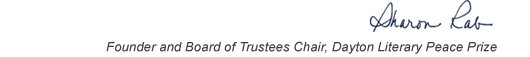table div table+table+table+table div table{width:100%;padding:0}table div table+table+table+table div table img{width:96.23%;padding:0;float:none}table div table+table+table+table div table td{width:100%;padding:0 1.88% 18px}/* styles */This list includes the winners and runners-up for 2015 and we have rich background information on each one on our website.

▪ Under Past Winners. scroll down to the year and click on it.
▪ You will have the option of clicking on a winner, a runner-up, or the finalists.
▪ If you click on the photo of winners and runners-up, you will find the author’s photo and bio, a selection from the book, the judges’ citation, the writer’s reflection on literature and peace, and the option of watching the introduction and acceptance speech.
 ▪ Under Past Winners. scroll down to the year and click on it.
 ▪ You will have the option of clicking on a winner, a runner-up, or the finalists.
 ▪ If you click on the photo of winners and runners-up, you will find the author’s photo and bio, a selection from the book, the judges’ citation, the writer’s reflection on literature and peace, and the option of watching the introduction and acceptance speech.

On the bottom right of the home page, you can click on COREScholar, which is built by the Chair of our Curriculum Committee Carol Loranger, Associate Dean of the College of Liberal Arts at Wright State, and maintained for us by Wright State University. There you can find interviews, TED Talks, articles, other books, films, etc. by and about each of our winning and runner-up authors. It is a great resource for students, book clubs, and readers who would like to explore an author in depth.

 table div table+table+table+table+table+table div table{width:100%;padding:0}table div table+table+table+table+table+table div table img{width:96.23%;padding:0;float:none}table div table+table+table+table+table+table div table td{width:100%;padding:0 1.88% 18px}/* styles *//* styles */ “Read global; buy local.” — Marlon James, DLPP 2009 Fiction Winner for The Book of Night Women
 table div table+table+table+table+table+table+table+table div table{width:100%;padding:0}table div table+table+table+table+table+table+table+table div table img{width:96.23%;padding:0;float:none}table div table+table+table+table+table+table+table+table div table td{width:100%;padding:0 1.88% 18px}/* styles */## 2015 Finalists - Fiction

 table div table+table+table+table+table+table+table+table+table+table div table{width:100%;padding:0}table div table+table+table+table+table+table+table+table+table+table div table img{width:96.23%;padding:0;float:none}table div table+table+table+table+table+table+table+table+table+table div table td{width:100%;padding:0 1.88% 18px}/* styles */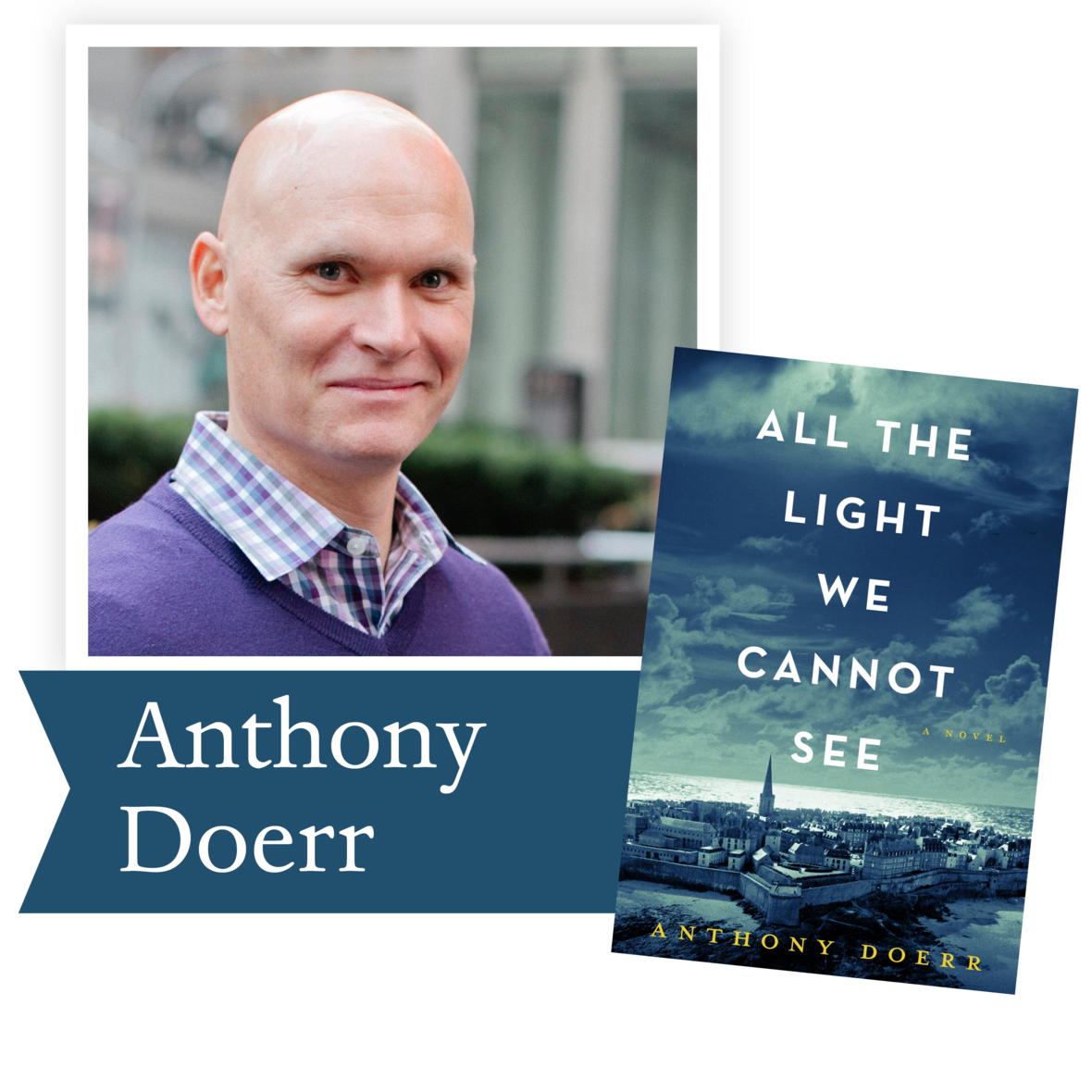All the Light We Cannot See by Anthony Doerr (Scribner): From the highly acclaimed Pulitzer Prize winner Anthony Doerr, a stunningly ambitious and beautiful novel about a blind French girl Marie-Laure and a
German boy Werner whose paths collide
in occupied France as they both try to
survive the devastation of World War II.

 table div table+table+table+table+table+table+table+table+table+table+table+table div table{width:100%;padding:0}table div table+table+table+table+table+table+table+table+table+table+table+table div table img{width:96.23%;padding:0;float:none}table div table+table+table+table+table+table+table+table+table+table+table+table div table td{width:100%;padding:0 1.88% 18px}/* styles */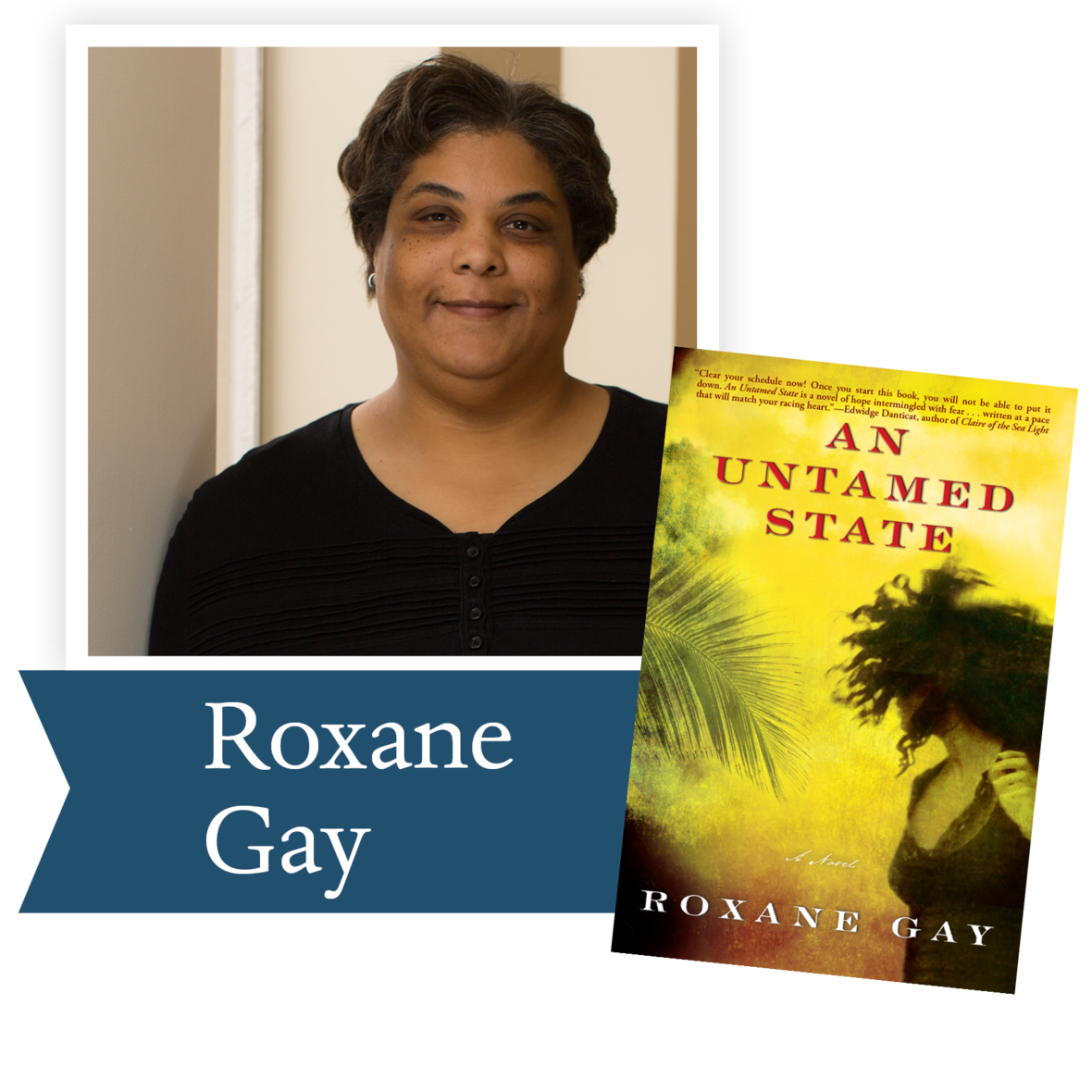An Untamed State by Roxane Gay (Grove Atlantic): Roxane Gay delivers an assured debut with a story of privilege in the face of crushing poverty, and of the lawless
anger that corrupt governments produce, finally showing us how redemption is found in the most unexpected of places.

 table div table+table+table+table+table+table+table+table+table+table+table+table+table+table div table{width:100%;padding:0}table div table+table+table+table+table+table+table+table+table+table+table+table+table+table div table img{width:96.23%;padding:0;float:none}table div table+table+table+table+table+table+table+table+table+table+table+table+table+table div table td{width:100%;padding:0 1.88% 18px}/* styles */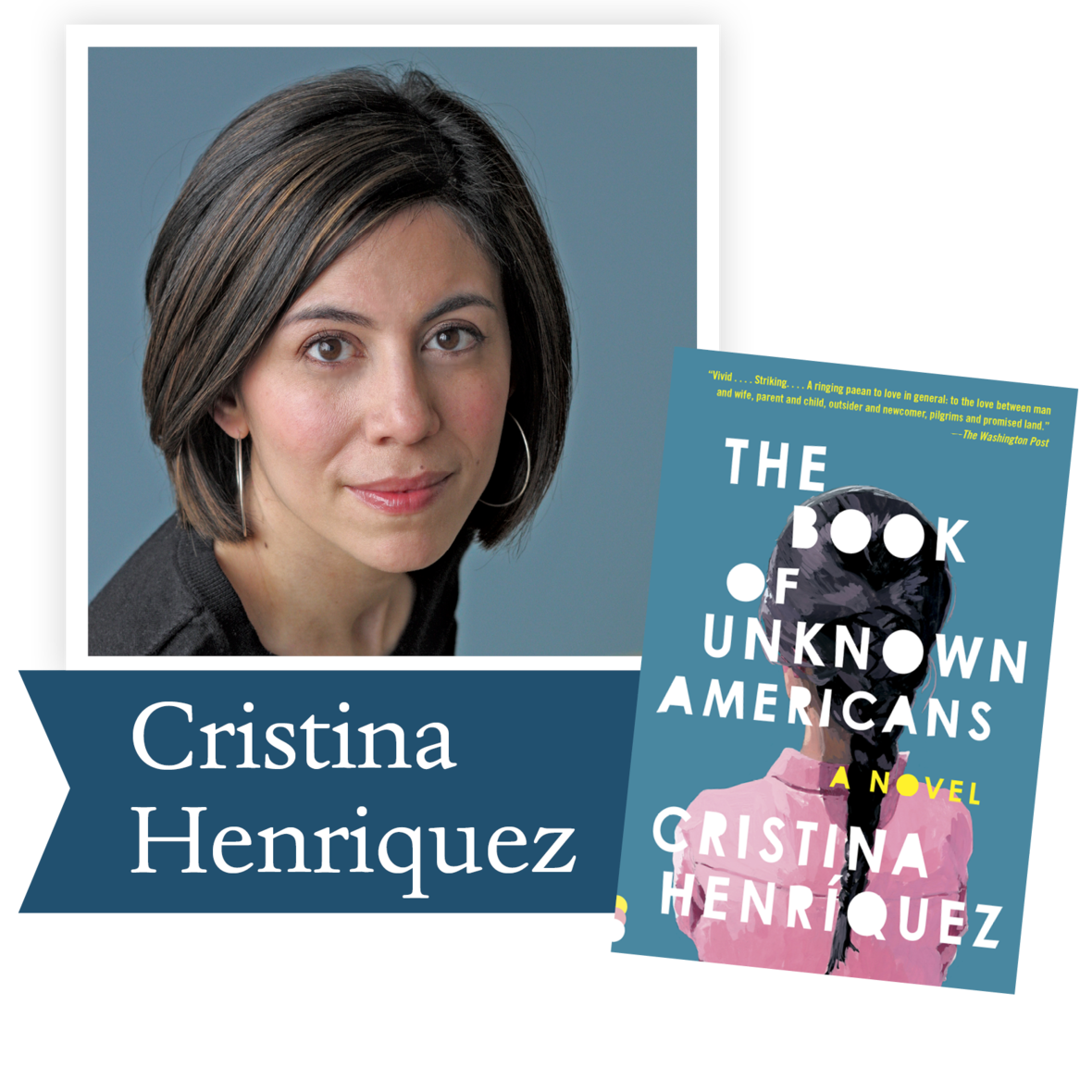The Book of Unknown Americans by Cristina Henríquez (Knopf): Henríquez seamlessly interweaves the story of two star-crossed lovers and their families with the testimonials of men and women who have come to the United States from all over Latin America in this novel of hopes and dreams, guilt, and love, offering a new definition of what it means to be American.

 table div table+table+table+table+table+table+table+table+table+table+table+table+table+table+table+table div table{width:100%;padding:0}table div table+table+table+table+table+table+table+table+table+table+table+table+table+table+table+table div table img{width:96.23%;padding:0;float:none}table div table+table+table+table+table+table+table+table+table+table+table+table+table+table+table+table div table td{width:100%;padding:0 1.88% 18px}/* styles */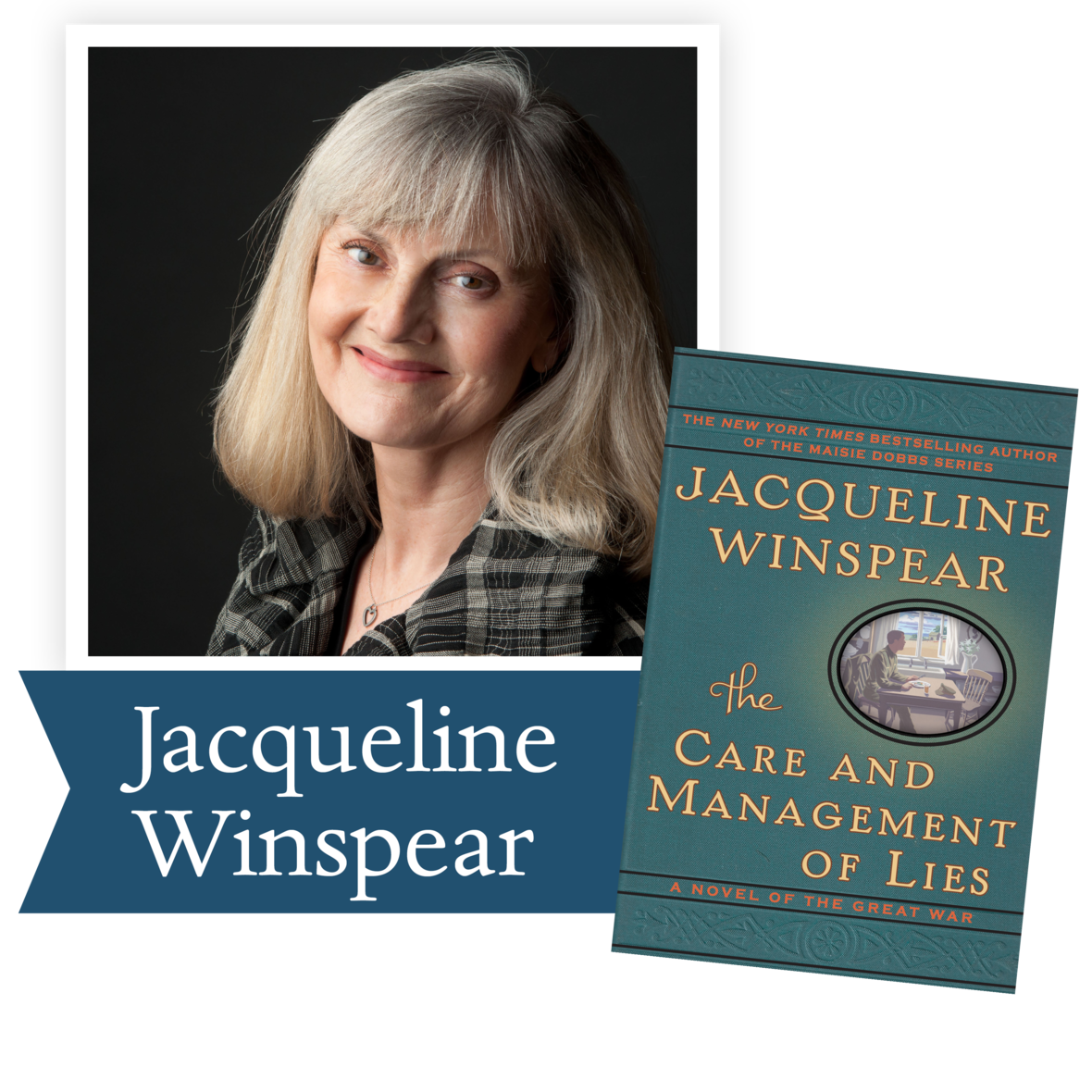The Care and Management of Lies by Jacqueline Winspear (HarperCollins): Set before and during the Great War, this is a tale of love and war in which a most unexpected player—food— becomes the ultimate expression of love, sharing center stage with blood-soaked trenches, home-front deprivation, and the changing roles of women. Raising profound questions about conflict, belief, and love that echo in our own time.

 table div table+table+table+table+table+table+table+table+table+table+table+table+table+table+table+table+table+table div table{width:100%;padding:0}table div table+table+table+table+table+table+table+table+table+table+table+table+table+table+table+table+table+table div table img{width:96.23%;padding:0;float:none}table div table+table+table+table+table+table+table+table+table+table+table+table+table+table+table+table+table+table div table td{width:100%;padding:0 1.88% 18px}/* styles */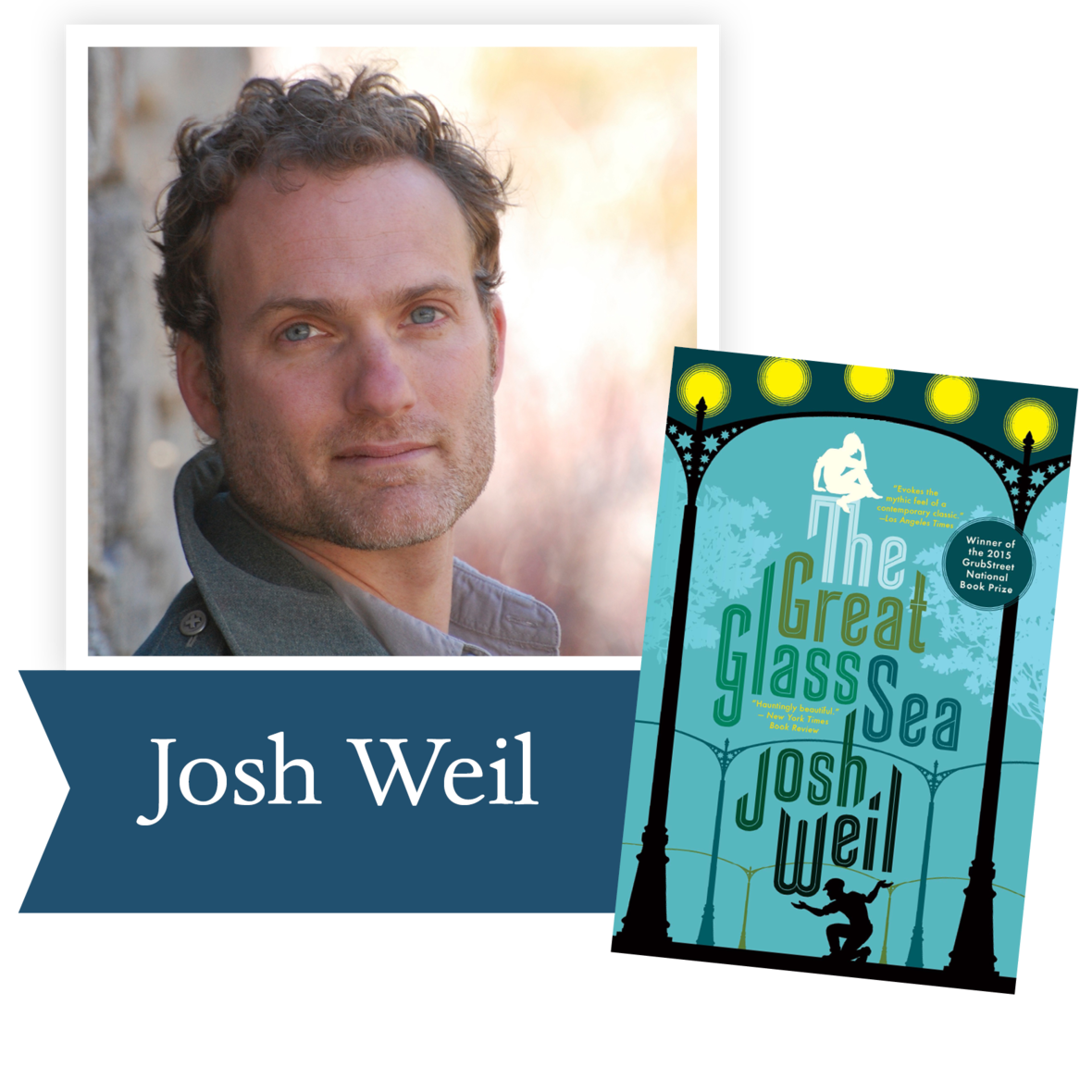The Great Glass Sea by Josh Weil (Grove Atlantic): This is an epic tragedy of brotherly
love set against the dystopian backdrop
of an alternative present-day Russia and swathed in all the magic of Russian folklore.

 table div table+table+table+table+table+table+table+table+table+table+table+table+table+table+table+table+table+table+table+table div table{width:100%;padding:0}table div table+table+table+table+table+table+table+table+table+table+table+table+table+table+table+table+table+table+table+table div table img{width:96.23%;padding:0;float:none}table div table+table+table+table+table+table+table+table+table+table+table+table+table+table+table+table+table+table+table+table div table td{width:100%;padding:0 1.88% 18px}/* styles */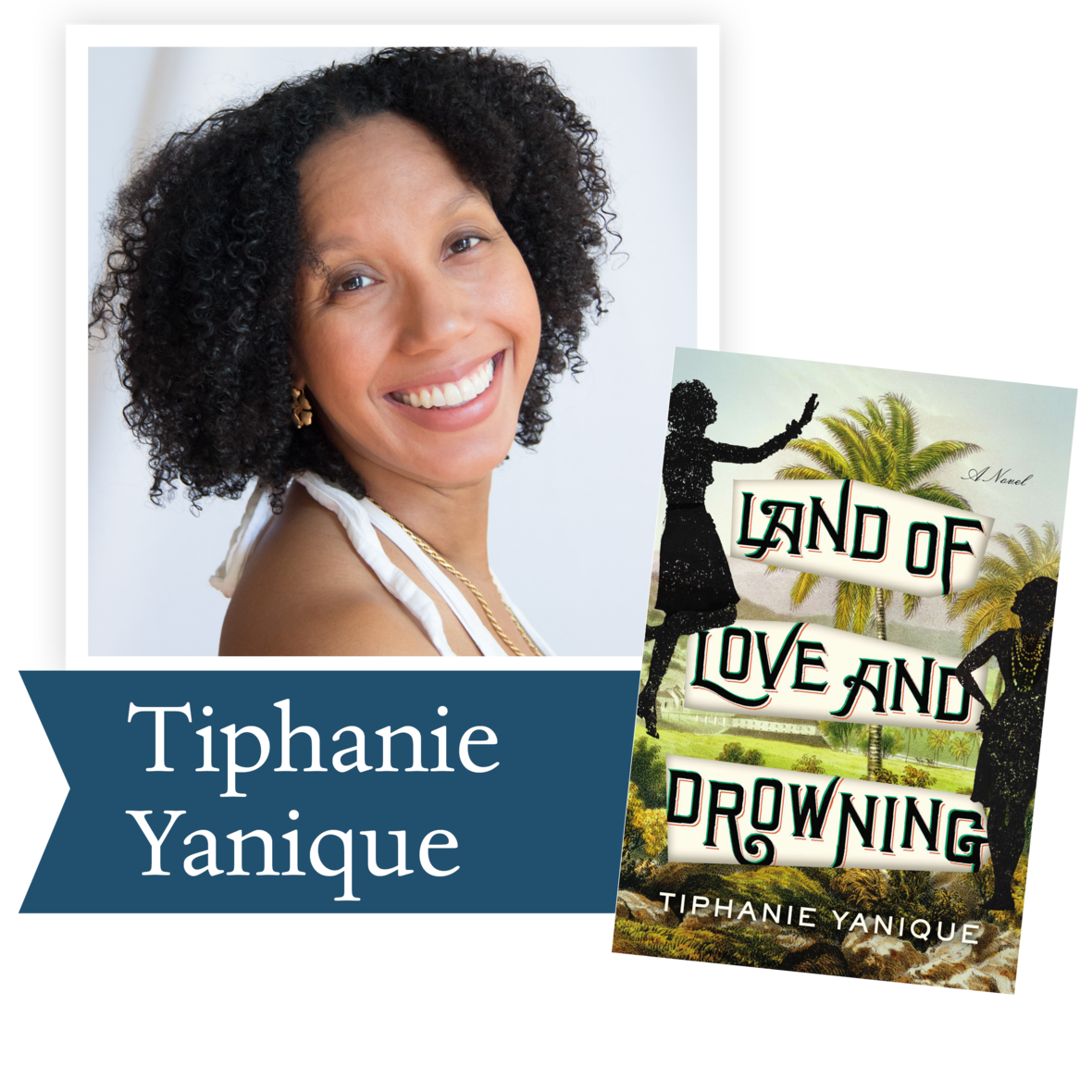Land of Love and Drowning by Tiphanie Yanique (Riverhead): Chronicling three generations of the Bradshaw family in the Virgin Islands, Land of Love and Drowning weaves together stories of magic and lust, unknown connections and hidden mysteries, family legacies, and an island world undergoing historical changes.

 table div table+table+table+table+table+table+table+table+table+table+table+table+table+table+table+table+table+table+table+table+table+table div table{width:100%;padding:0}table div table+table+table+table+table+table+table+table+table+table+table+table+table+table+table+table+table+table+table+table+table+table div table img{width:96.23%;padding:0;float:none}table div table+table+table+table+table+table+table+table+table+table+table+table+table+table+table+table+table+table+table+table+table+table div table td{width:100%;padding:0 1.88% 18px}/* styles */## 2015 Finalists - Nonfiction

 table div table+table+table+table+table+table+table+table+table+table+table+table+table+table+table+table+table+table+table+table+table+table+table+table div table{width:100%;padding:0}table div table+table+table+table+table+table+table+table+table+table+table+table+table+table+table+table+table+table+table+table+table+table+table+table div table img{width:96.23%;padding:0;float:none}table div table+table+table+table+table+table+table+table+table+table+table+table+table+table+table+table+table+table+table+table+table+table+table+table div table td{width:100%;padding:0 1.88% 18px}/* styles */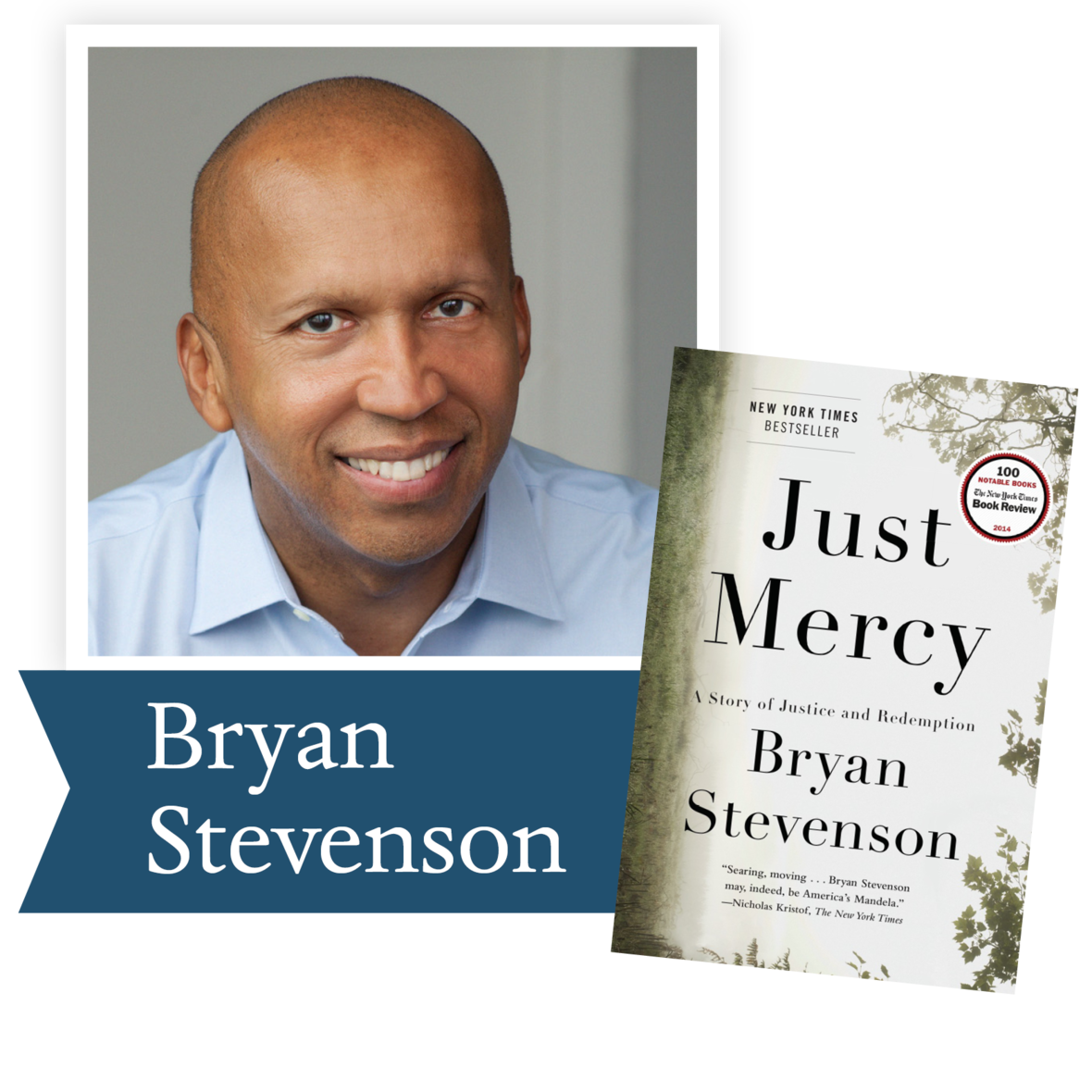Just Mercy by Bryan Stevenson (Spiegel and Grau): From one of the country’s most visionary legal thinkers, social justice advocates, and MacArthur “geniuses,” this is an intimate and unforgettable narrative journey into the broken American criminal justice system, an exquisitely rendered account of a heroic advocate’s fights on behalf of the most powerless people in our society.

 table div table+table+table+table+table+table+table+table+table+table+table+table+table+table+table+table+table+table+table+table+table+table+table+table+table+table div table{width:100%;padding:0}table div table+table+table+table+table+table+table+table+table+table+table+table+table+table+table+table+table+table+table+table+table+table+table+table+table+table div table img{width:96.23%;padding:0;float:none}table div table+table+table+table+table+table+table+table+table+table+table+table+table+table+table+table+table+table+table+table+table+table+table+table+table+table div table td{width:100%;padding:0 1.88% 18px}/* styles */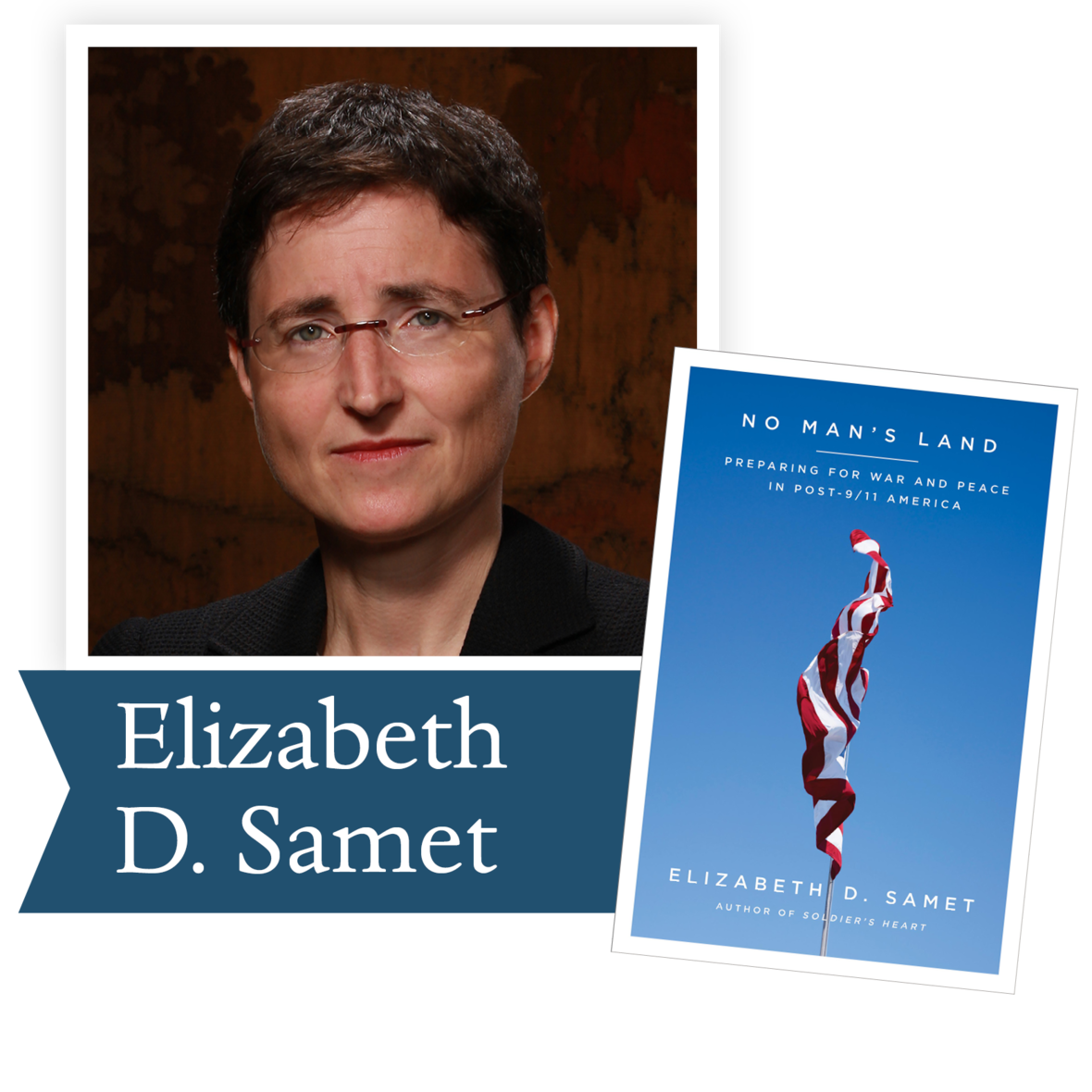No Man’s Land by Elizabeth D. Samet
(Farrar, Straus and Giroux): This book offers a moving, urgent examination of what it means to negotiate the tensions between soldier and civilian, between war and peace, between “over there” and “over here”— between life on the front and life at home.

 table div table+table+table+table+table+table+table+table+table+table+table+table+table+table+table+table+table+table+table+table+table+table+table+table+table+table+table+table div table{width:100%;padding:0}table div table+table+table+table+table+table+table+table+table+table+table+table+table+table+table+table+table+table+table+table+table+table+table+table+table+table+table+table div table img{width:96.23%;padding:0;float:none}table div table+table+table+table+table+table+table+table+table+table+table+table+table+table+table+table+table+table+table+table+table+table+table+table+table+table+table+table div table td{width:100%;padding:0 1.88% 18px}/* styles */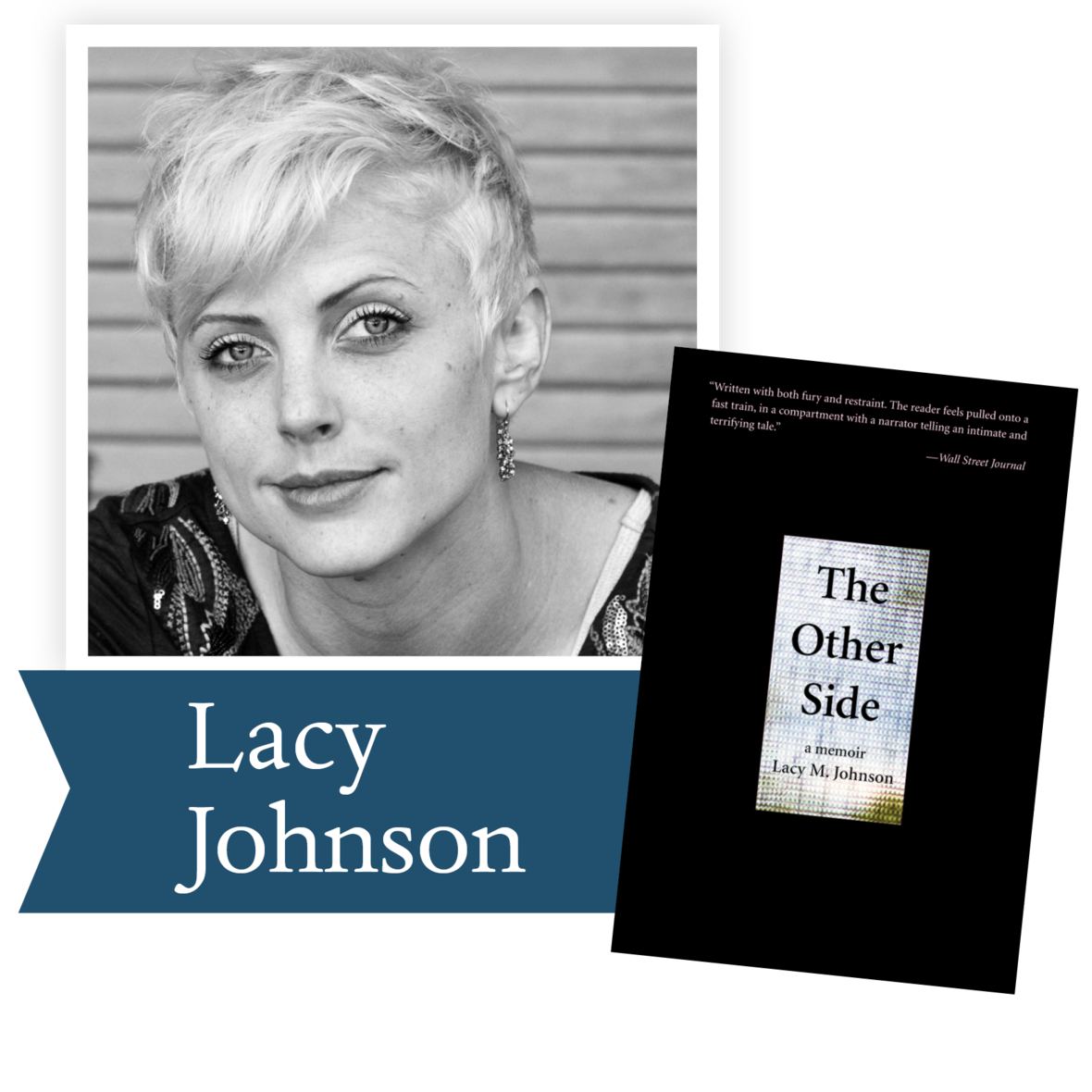The Other Side by Lacy Johnson (Tin House Books): This is the haunting account of a first passionate and then abusive relationship, the events leading to Johnson’s kidnapping and imprisonment, her dramatic escape, and her hard-fought struggle to recover, raising timely questions about gender roles and the epidemic of violence against women.

 table div table+table+table+table+table+table+table+table+table+table+table+table+table+table+table+table+table+table+table+table+table+table+table+table+table+table+table+table+table+table div table{width:100%;padding:0}table div table+table+table+table+table+table+table+table+table+table+table+table+table+table+table+table+table+table+table+table+table+table+table+table+table+table+table+table+table+table div table img{width:96.23%;padding:0;float:none}table div table+table+table+table+table+table+table+table+table+table+table+table+table+table+table+table+table+table+table+table+table+table+table+table+table+table+table+table+table+table div table td{width:100%;padding:0 1.88% 18px}/* styles */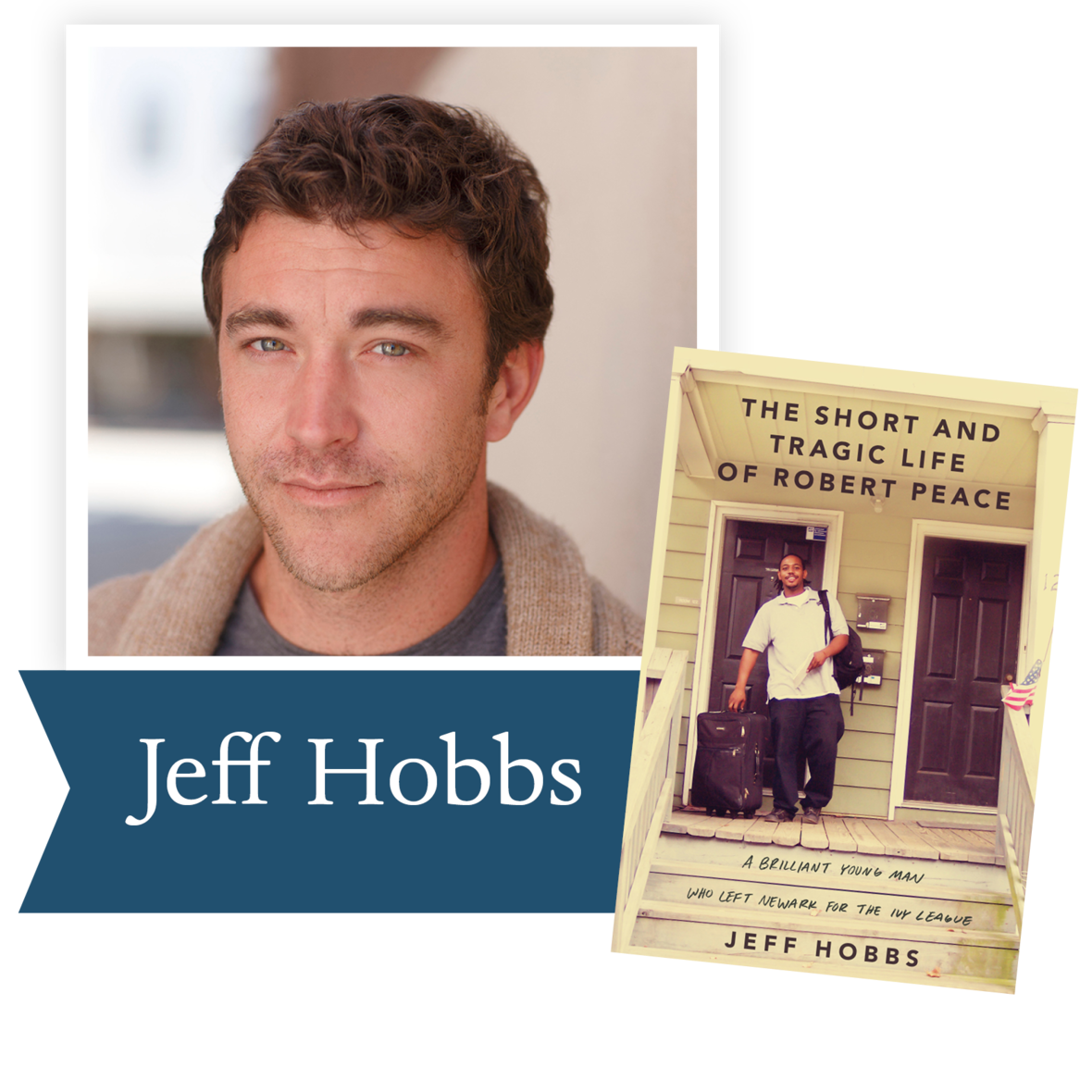The Short and Tragic Life of Robert
Peace by Jeff Hobbs (Scribner): Written by his college roommate, The Short and Tragic Life of Robert Peace is the brilliant, deeply-researched account of the life of Robert DeShaun Peace, a talented young African-American man who left the ghettos of Newark, New Jersey, on a full scholarship to Yale University, but who was tragically murdered in a basement marijuana lab after he graduated.

 table div table+table+table+table+table+table+table+table+table+table+table+table+table+table+table+table+table+table+table+table+table+table+table+table+table+table+table+table+table+table+table+table div table{width:100%;padding:0}table div table+table+table+table+table+table+table+table+table+table+table+table+table+table+table+table+table+table+table+table+table+table+table+table+table+table+table+table+table+table+table+table div table img{width:96.23%;padding:0;float:none}table div table+table+table+table+table+table+table+table+table+table+table+table+table+table+table+table+table+table+table+table+table+table+table+table+table+table+table+table+table+table+table+table div table td{width:100%;padding:0 1.88% 18px}/* styles */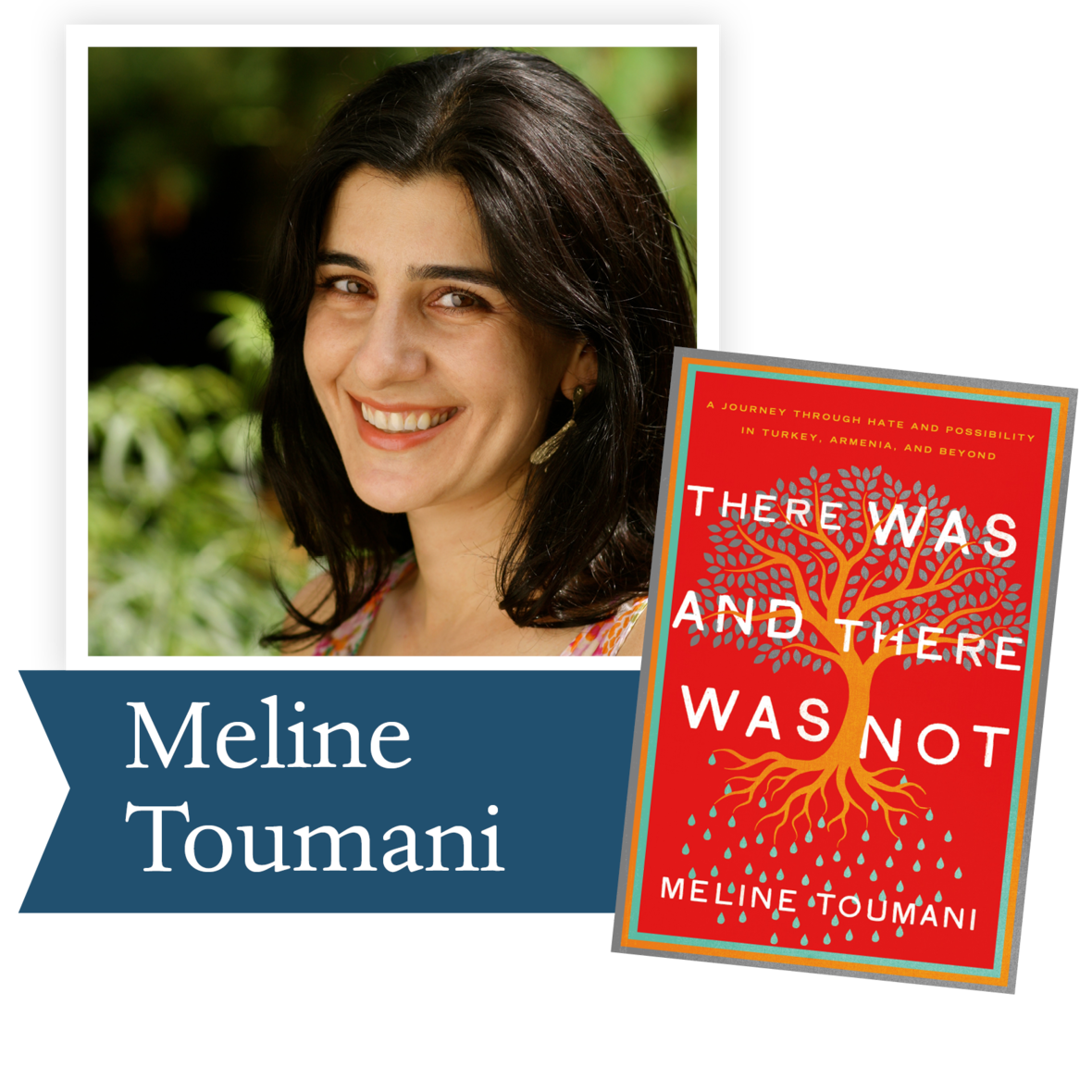There Was and There Was Not by Meline Toumani (Metropolitan Books): Frustrated by the all-consuming nature of her close-knit Armenian community’s quest for genocide recognition by Turkey, Toumani moved to Istanbul. This account of her “love thine enemy” experiment probes universal questions: how to belong to a community without conforming to it, how to acknowledge a tragedy without exploiting it, and, most important, how to remember a genocide without perpetuating the kind of hatred that makes such atrocities possible in the first place.

 table div table+table+table+table+table+table+table+table+table+table+table+table+table+table+table+table+table+table+table+table+table+table+table+table+table+table+table+table+table+table+table+table+table+table div table{width:100%;padding:0}table div table+table+table+table+table+table+table+table+table+table+table+table+table+table+table+table+table+table+table+table+table+table+table+table+table+table+table+table+table+table+table+table+table+table div table img{width:96.23%;padding:0;float:none}table div table+table+table+table+table+table+table+table+table+table+table+table+table+table+table+table+table+table+table+table+table+table+table+table+table+table+table+table+table+table+table+table+table+table div table td{width:100%;padding:0 1.88% 18px}/* styles */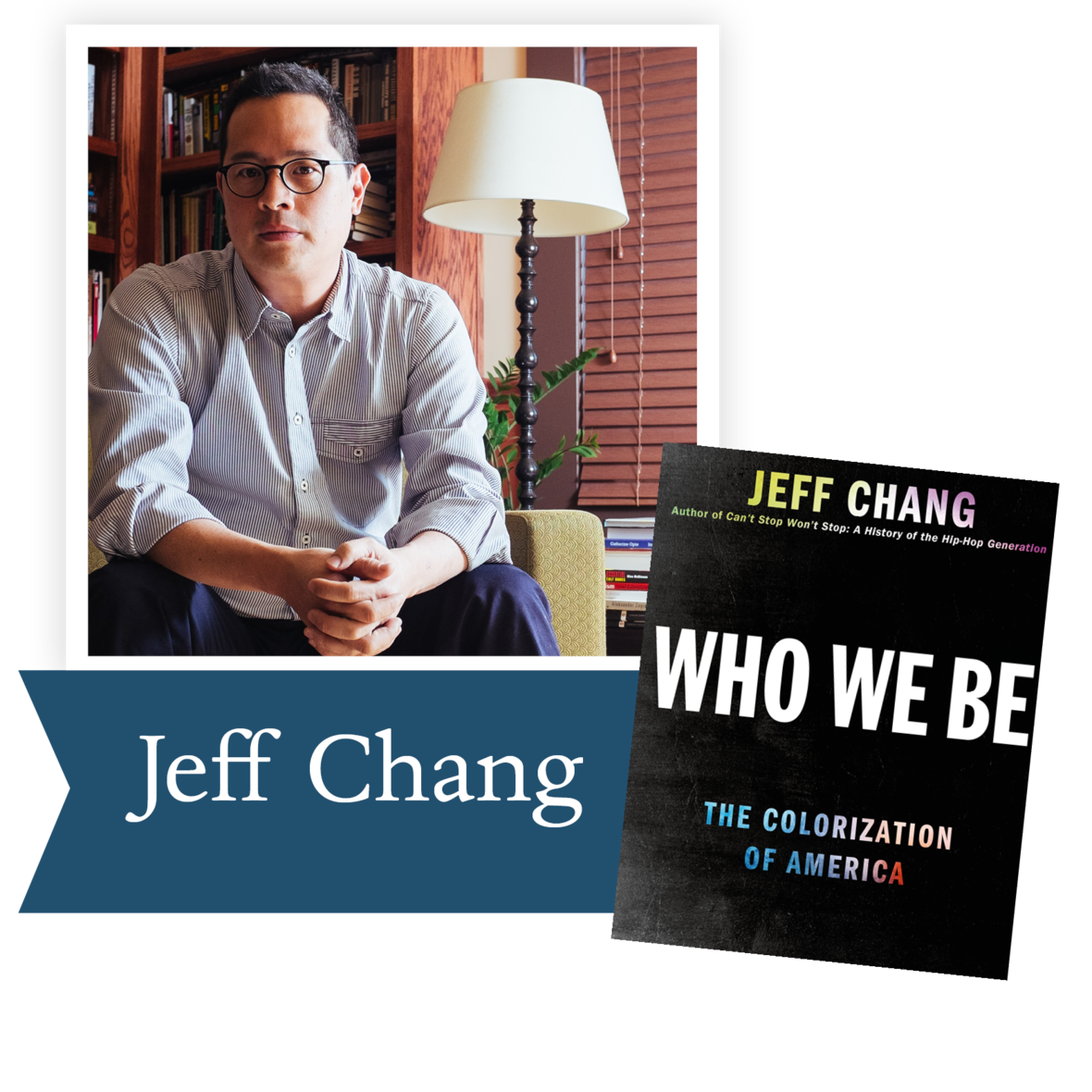Who We Be by Jeff Chang (St. Martin’s Press): This book explores the changing (and unchanging) ways that the U.S. has viewed race over the past half-century, asking whether or not, in the eras of “multicultural” and “post-racial” cultures, we really see each other more clearly.

 table div table+table+table+table+table+table+table+table+table+table+table+table+table+table+table+table+table+table+table+table+table+table+table+table+table+table+table+table+table+table+table+table+table+table+table+table div table{width:100%;padding:0}table div table+table+table+table+table+table+table+table+table+table+table+table+table+table+table+table+table+table+table+table+table+table+table+table+table+table+table+table+table+table+table+table+table+table+table+table div table img{width:96.23%;padding:0;float:none}table div table+table+table+table+table+table+table+table+table+table+table+table+table+table+table+table+table+table+table+table+table+table+table+table+table+table+table+table+table+table+table+table+table+table+table+table div table td{width:100%;padding:0 1.88% 18px}/* styles */## 2015 Ambassador Richard C. Holbrooke Distinguished Achievement Award Winner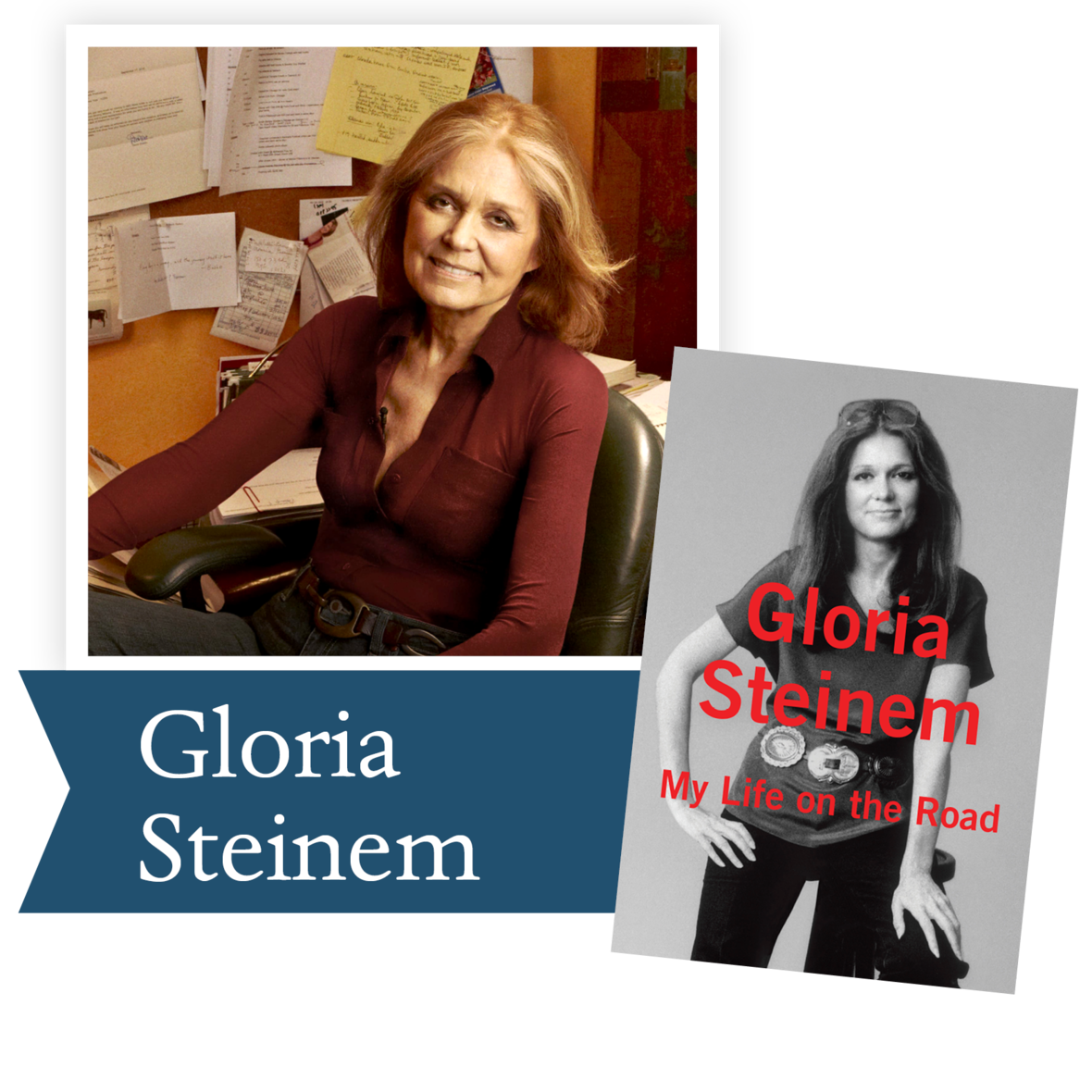Gloria Steinem’s My Life on the Road follows the path of how a nomadic girl from Toledo became a leading feminist in the quest for equality. Here in Dayton, she inspired us to continue to recognize that there cannot be peace until women are safe in their own homes, on the streets, and in the workplace. She has preached nonviolence and has protested the caste system in India, the civil rights movement, the fair treatment of farm workers, the protection of the environment and the end of war. If you are not familiar with the life and work of Gloria Steinem, My Life on the Road will give you insight into the evolution of one of the seminal figures of our time.

We invite you to read the New York Times review of My Life on the Road.

 table div table+table+table+table+table+table+table+table+table+table+table+table+table+table+table+table+table+table+table+table+table+table+table+table+table+table+table+table+table+table+table+table+table+table+table+table+table+table+table div table{width:100%;padding:0}table div table+table+table+table+table+table+table+table+table+table+table+table+table+table+table+table+table+table+table+table+table+table+table+table+table+table+table+table+table+table+table+table+table+table+table+table+table+table+table div table img{width:96.23%;padding:0;float:none}table div table+table+table+table+table+table+table+table+table+table+table+table+table+table+table+table+table+table+table+table+table+table+table+table+table+table+table+table+table+table+table+table+table+table+table+table+table+table+table div table td{width:100%;padding:0 1.88% 18px}/* styles */table div table+table+table+table+table+table+table+table+table+table+table+table+table+table+table+table+table+table+table+table+table+table+table+table+table+table+table+table+table+table+table+table+table+table+table+table+table+table+table+table+table div table{width:100%;padding:0}table div table+table+table+table+table+table+table+table+table+table+table+table+table+table+table+table+table+table+table+table+table+table+table+table+table+table+table+table+table+table+table+table+table+table+table+table+table+table+table+table+table div table img{width:96.23%;padding:0;float:none}table div table+table+table+table+table+table+table+table+table+table+table+table+table+table+table+table+table+table+table+table+table+table+table+table+table+table+table+table+table+table+table+table+table+table+table+table+table+table+table+table+table div table td{width:100%;padding:0 1.88% 18px}/* styles */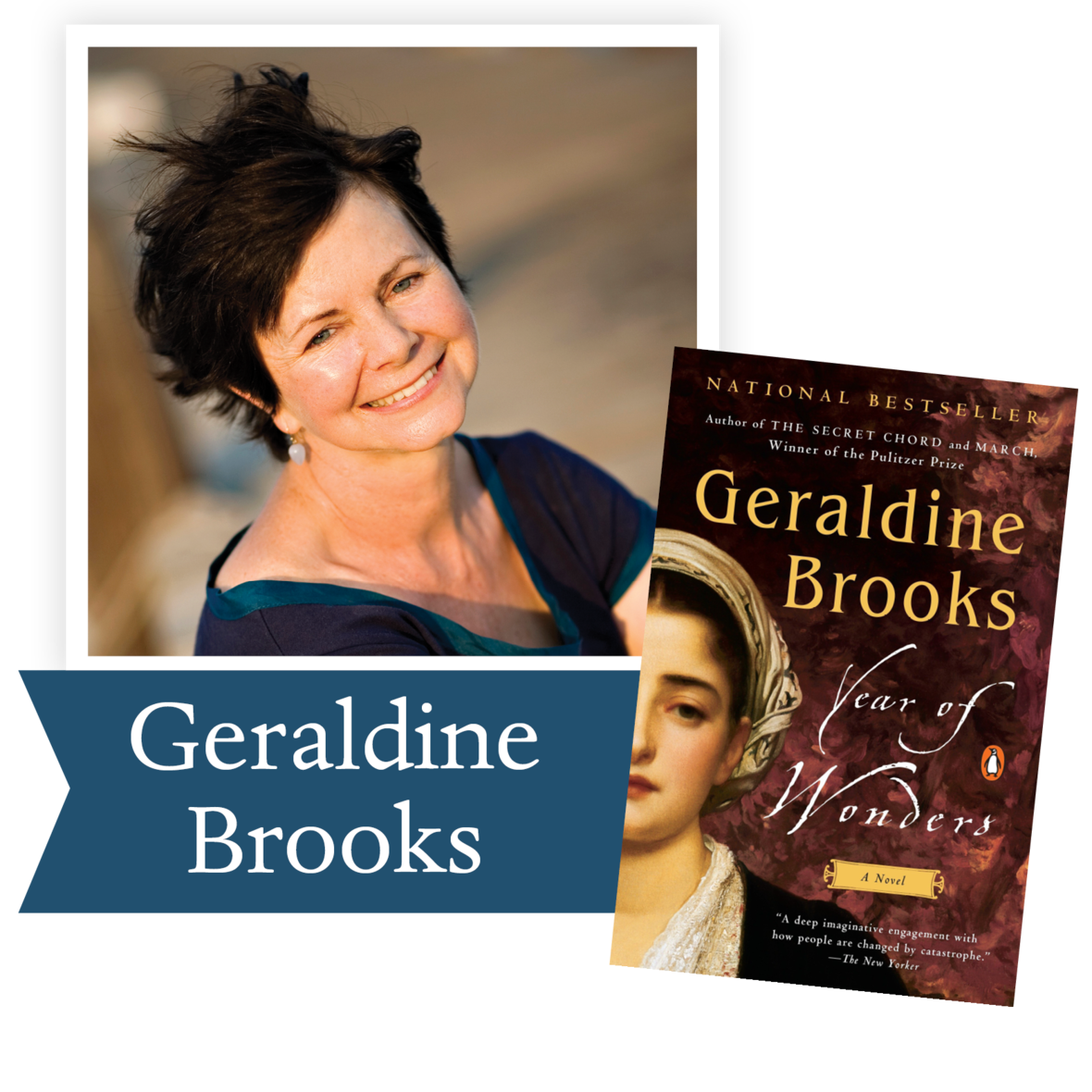“Plague stories remind us that we cannot manage without community . . . Year of Wonders is a testament to that very notion.” – The Washington Post

An unforgettable tale, set in 17th century England, of a village that quarantines itself to arrest the spread of the plague, from the author of The Secret Chord and of March, winner of the Pulitzer Prize.

Ron Rollins, Dayton Daily News Ideas and Voices Editor, will moderate the discussion of The Year of Wonders.

Email Emily Kretzer to be added to the list. We'll send details as they become available.

 table div table+table+table+table+table+table+table+table+table+table+table+table+table+table+table+table+table+table+table+table+table+table+table+table+table+table+table+table+table+table+table+table+table+table+table+table+table+table+table+table+table+table+table div table{width:100%;padding:0}table div table+table+table+table+table+table+table+table+table+table+table+table+table+table+table+table+table+table+table+table+table+table+table+table+table+table+table+table+table+table+table+table+table+table+table+table+table+table+table+table+table+table+table div table img{width:96.23%;padding:0;float:none}table div table+table+table+table+table+table+table+table+table+table+table+table+table+table+table+table+table+table+table+table+table+table+table+table+table+table+table+table+table+table+table+table+table+table+table+table+table+table+table+table+table+table+table div table td{width:100%;padding:0 1.88% 18px}/* styles */Support the Dayton Literary Peace Prize when you shop with Amazon using this link.